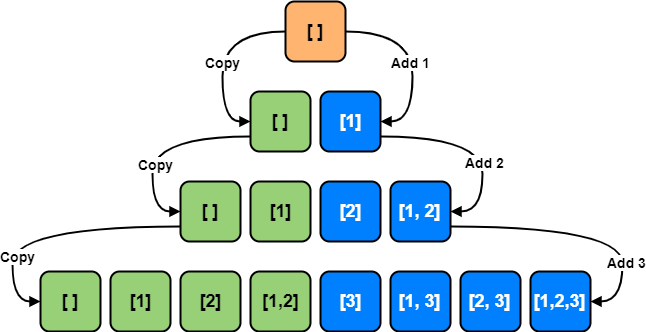# Coding Patterns: Subsets

In Coding Patterns series, we will try to recognize common patterns underlying behind each algorithm question, using real examples from Leetcode.

Previous posts were about Sliding Window, Two Pointers, Fast & Slow Pointers, Merge Intervals, Cyclic Sort, In-place Reversal of a Linked List, Breadth First Search (BFS), Depth First Search (DFS) and Two Heaps patterns and today, we will introduce Subsets pattern which is very useful to solve the problems involve dealing with Permutations and Combinations of a given set of elements.

This pattern describes an efficient Breadth First Search (BFS) approach to handle all these problems.

## Problem: Subsets

LeetCode 78 - Subsets [medium]

Given a set of distinct integers, `nums`, return all possible subsets (the power set).

Note: The solution set must not contain duplicate subsets.

Example:

``````Input: nums = [1, 2, 3]
Output:
[
,
,
,
[1, 2, 3],
[1, 3],
[2, 3],
[1, 2],
[]
]
``````

### Subsets Solution

To generate all possible subsets, we can use the Breadth First Search (BFS) approach. Starting with an empty set, we will iterate through all numbers one-by-one, and add them to existing sets to create subsets.

2. Add `num` (1) to existing sets: [[ ], ]
3. Add `num` (2) to existing sets: [[ ], , , [1, 2]]
4. Add `num` (3) to existing sets: [[ ], , , [1, 2], , [1, 3], [2, 3], [1, 2, 3]]``````from typing import List

class Solution:
def subsets(self, nums: List[int]) -> List[List[int]]:
subsets = [[]]

for num in nums:
for i in range(len(subsets)):
subsets.append(subsets[i] + [num])
return subsets
``````

Time Complexity: O(2N) since, in each step, number of subsets doubles.

Space Complexity: O(2N)

## How to identify?

If the problem description involves dealing with Permutations and Combinations of a given set of elements or subsets, you should think about Subsets pattern which is very useful to solve these kinds of problems.

This pattern describes an efficient Breadth First Search (BFS) approach to handle all these problems.

Tags:

Categories:

Updated: